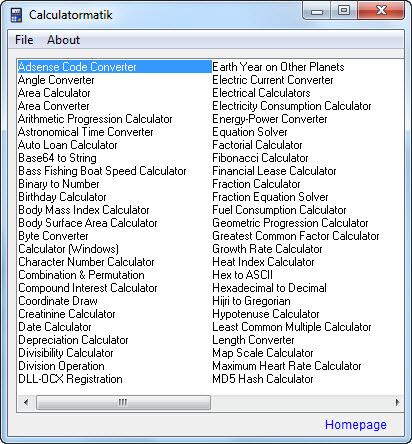# Calculatormatik – calculator and converter

Calculatormatik is a free windows software that includes many calculators and converters.

It includes:

Angle Converter
Area Calculator
Area Converter
Arithmetic Progression Calculator
Astronomical Time Converter
Auto Loan Calculator
Base64 to String
Bass Fishing Boat Speed Calculator
Binary to Number
Birthday Calculator
Body Mass Index Calculator
Body Surface Area Calculator
Byte Converter
Calculator (Windows)
Character Number Calculator
Combination & Permutation Calculator
Compound Interest Calculator
Coordinate Draw
and moreComplete calculators and converters list: